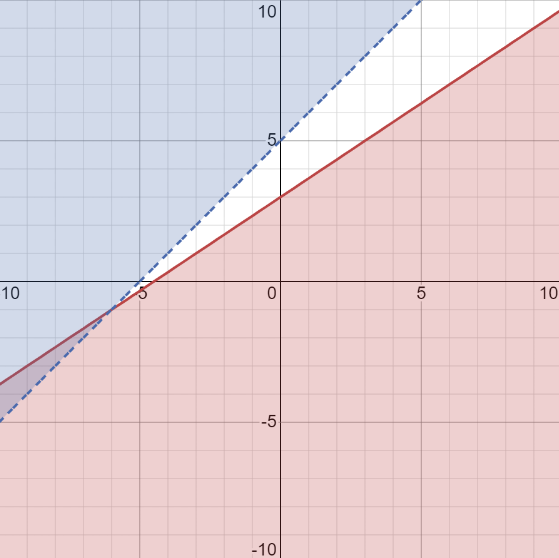Axel123

2022-01-10

Graph the system of inequalities below, and clearly label the solution set y≤-2/3x+3 y>x+5nick1337

Graph $y\le -\frac{2}{3}x+3$

Find the slope and the y-intercept for the boundary line.

Slope: $\frac{2}{3}$

y-intercept: $\left(0,3\right)$

Graph a solid line, then shade the area below the boundary line since $y$ is less

than $\frac{2}{3}x+3$

Graph $y>x+5$

Use the slope-intercept form to find the slope and y-intercept.

Slope: $1$

y-intercept: $\left(0,5\right)$

Graph a dashed line, then shade the area above the boundary line since $y$ is greater than $x+5$

Plot each graph on the same coordinate system.Do you have a similar question?